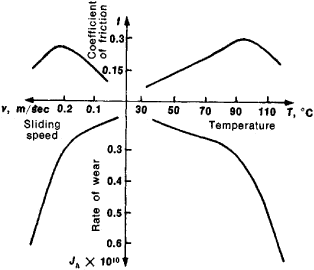# friction measurement

The following article is from The Great Soviet Encyclopedia (1979). It might be outdated or ideologically biased.

## Friction Measurement

the measurement of the force or coefficient of friction, the limiting friction, and the extent of wear of rubbing surfaces. Friction measurements are divided into the following two types: laboratory measurements, in which the frictional forces and wear resistance of materials under various conditions are evaluated, and field measurements, in which a given friction unit is evaluated as a whole.

In laboratory tests, one uses specimens that are in point or line contact, such as a sphere on a plane or two crossed cylinders rubbing along a generatrix, and specimens that have small areas of contact, such as a ball in a spherical hollow, a pin with its end touching a disk, or two abutting cylinders. With these specimens it is possible to obtain the frictional force and the wear per unit of actual contact. These quantities can be used to calculate the frictional force and wear for any area of the surface. Gauges containing elastic elements are ordinarily used to measure frictional force.

The evaluation of friction pairs makes it possible to obtain a series of successive values of the frictional force and wear under conditions of friction that gradually increase in severity, that is, as the sliding speed or load, which have different effects, is increased. The load increases the number of the points of contact without changing their quality and leads to a change in bulk heating. The sliding speed, by increasing the temperature at any one point of contact, results in qualitative changes in the contact and alters the temperature gradient through the material. Curves of frictional heat stability, that is, the temperature dependence of the coefficient of friction and the rate of wear (see Figure 1) are the most important characteristics of a friction pair; the curves are obtained when two circular cylindrical specimens are subjected to endwise friction under a constant load with a stepwise increasing sliding speed, which provides a stepwise change in temperature. The temperatures are measured with a thermocouple embedded in one of the specimens. The rate of wear is estimated from the dimensionless ratio of the thickness of the worn layer and the distance covered by sliding.Figure 1. Curve of frictional heat stability for a friction pair consisting of a plastic composite and steel at a pressure of 150 × 104 new-tons per m2

The limiting friction is estimated by letting a given friction pair seize—that is, by allowing the frictional force to increase sharply and the contact surface to be damaged while smoothly changing the sliding speed or load. The results of laboratory tests are applied to real friction pairs by taking into account the relations of similarity theory.

The frictional force in real machines is measured by various methods, for example, by using a measurement of the power consumed during idling or by employing gauges that measure, from the angle of torsion of a shaft, the magnitude of the friction torque or the frictional force. Measurement of the temperatures of a friction unit makes it possible to estimate the frictional force by computation; such measurements provide an indirect but very convenient method of evaluating friction. The coefficient of rolling resistance is determined by means of traction dynamometers.

### REFERENCE

Chichinadze, A. V. Raschet i issledovanie vneshnego treniia pri tormozhenii. Moscow, 1967.

I. V. KRAGEL’SKII

## friction measurement

A measurement of the friction characteristics of the runway pavement surface using continuous self-watering friction measurement equipment in accordance with AC 150/5320–12 Measurement, Construction, and Maintenance of Skid Resistant Airport Pavement Surfaces.